# Contents

Most of the pipeline and visualizations presented herein have been adapted from Nowicka et al. (2019)’s “CyTOF workflow: differential discovery in high-throughput high-dimensional cytometry datasets” available here.

# load required packages
library(CATALYST)
library(cowplot)
library(flowCore)
library(diffcyt)
library(scater)
library(SingleCellExperiment)

# 1 Example data

• PBMC_fs:
a flowSet holding PBMCs samples from 4 patients, each containing between 500 and 1000 cells. For each sample, the expression of 10 cell surface and 14 signaling markers was measured before (REF) and upon BCR/FcR-XL stimulation (BCRXL) with B cell receptor/Fc receptor crosslinking for 30’, resulting in a total of 8 samples.
This data set represents a subset of data originating from Bodenmiller et al. (2012) that was also used in the citrus paper (Bruggner et al. 2014).
• PBMC_panel:
a data.frame containing each marker’s column name in the FCS file (fcs_colname column), its targeted protein marker (antigen column), and the marker_class (“type” or “state”).
• PBMC_md:
a data.frame where rows correspond to samples, and columns specify each sample’s file_name, sample_id, condition, and patient_id.
# load example data
data(PBMC_fs, PBMC_panel, PBMC_md)
PBMC_fs
## A flowSet with 8 experiments.
##
## column names(24): CD3(110:114)Dd CD45(In115)Dd ... HLA-DR(Yb174)Dd
##   CD7(Yb176)Dd
head(PBMC_panel)
##      fcs_colname antigen marker_class
## 1 CD3(110:114)Dd     CD3         type
## 2  CD45(In115)Dd    CD45         type
## 3 pNFkB(Nd142)Dd   pNFkB        state
## 4  pp38(Nd144)Dd    pp38        state
## 5   CD4(Nd145)Dd     CD4         type
## 6  CD20(Sm147)Dd    CD20         type
head(PBMC_md)
##                 file_name sample_id condition patient_id
## 1 PBMC_patient1_BCRXL.fcs    BCRXL1     BCRXL   Patient1
## 2   PBMC_patient1_Ref.fcs      Ref1       Ref   Patient1
## 3 PBMC_patient2_BCRXL.fcs    BCRXL2     BCRXL   Patient2
## 4   PBMC_patient2_Ref.fcs      Ref2       Ref   Patient2
## 5 PBMC_patient3_BCRXL.fcs    BCRXL3     BCRXL   Patient3
## 6   PBMC_patient3_Ref.fcs      Ref3       Ref   Patient3

The code snippet below demonstrates how to construct a flowSet from a set of FCS files. However, we also give the option to directly specify the path to a set of FCS files (see next section).

# download exemplary set of FCS files
url <- "http://imlspenticton.uzh.ch/robinson_lab/cytofWorkflow"
zip <- "PBMC8_fcs_files.zip"
unzip(zip)

# read in FCS files as flowSet
fcs <- list.files(pattern = ".fcs$") fs <- read.flowSet(fcs, transformation = FALSE, truncate_max_range = FALSE) # 2 Data preparation Data used and returned throughout differential analysis are held in objects of the SingleCellExperiment class. To bring the data into the appropriate format, prepData() requires the following inputs: • x: a flowSet holding the raw measurement data, or a character string that specifies a path to a set of FCS files. • panel: a 2 column data.frame that contains for each marker of interest i) its column name in the raw input data, and ii) its targeted protein marker. • md: a data.frame with columns describing the experimental design. Optionally, features will specify which columns (channels) to keep from the input data. Here, we keep all measurement parameters (default value features = NULL). (sce <- prepData(PBMC_fs, PBMC_panel, PBMC_md)) ## class: SingleCellExperiment ## dim: 24 5428 ## metadata(2): experiment_info chs_by_fcs ## assays(2): counts exprs ## rownames(24): CD3 CD45 ... HLA-DR CD7 ## rowData names(3): channel_name marker_name marker_class ## colnames: NULL ## colData names(3): sample_id condition patient_id ## reducedDimNames(0): ## mainExpName: NULL ## altExpNames(0): We provide flexibility in the way the panel and metadata table can be set up. Specifically, column names are allowed to differ from the example above, and multiple factors (patient ID, conditions, batch etc.) can be specified. Arguments panel_cols and md_cols should then be used to specify which columns hold the required information. An example is given below: # alter panel column names panel2 <- PBMC_panel colnames(panel2)[1:2] <- c("channel_name", "marker") # alter metadata column names & add 2nd condition md2 <- PBMC_md colnames(md2) <- c("file", "sampleID", "cond1", "patientID") md2$cond2 <- rep(c("A", "B"), 4)

# construct SCE
prepData(PBMC_fs, panel2, md2,
panel_cols = list(channel = "channel_name", antigen = "marker"),
md_cols = list(file = "file", id = "sampleID",
factors = c("cond1", "cond2", "patientID")))

Note that, independent of the input panel and metadata tables, the constructor will fix the names of mandatory slots for latter data accession (sample_id in the rowData, channel_name and marker_name in the colData). The md table will be stored under experiment_info inside the metadata.

# 3 Clustering

CATALYST provides a simple wrapper to perform high resolution FlowSOM clustering and lower resolution ConsensusClusterPlus metaclustering. By default, the data will be initially clustered into xdim = 10 x ydim = 10 = 100 groups. Secondly, the function will metacluster populations into 2 through maxK (default 20) clusters. To make analyses reproducible, the random seed may be set via seed. By default, if the colData(sce)$marker_class column is specified, the set of markers with marker class "type" will be used for clustering (argument features = "type"). Alternatively, the markers that should be used for clustering can be specified manually. sce <- cluster(sce, features = "type", xdim = 10, ydim = 10, maxK = 20, verbose = FALSE, seed = 1)  Let K = xdim x ydim be the number of FlowSOM clusters. cluster will add information to the following slots of the input SingleCellExperiment: • rowData: • cluster_id: cluster ID as inferred by FlowSOM. One of 1, …, K. • colData: • marker_class: factor "type" or "state". Specifyies whether a marker has been used for clustering or not, respectively. • metadata: • SOM_codes: a table with dimensions K x (# type markers). Contains the SOM codes. • cluster_codes: a table with dimensions K x (maxK + 1). Contains the cluster codes for all metaclusterings. • delta_area: a ggplot object (see below for details). ## 3.2mergeClusters: Manual cluster merging Provided with a 2 column data.frame containing old_cluster and new_cluster IDs, mergeClusters allows for manual cluster merging of any clustering available within the input SingleCellExperiment (i.e. the xdim x ydim FlowSOM clusters, and any of the 2-maxK ConsensusClusterPlus metaclusters). For latter accession (visualization, differential testing), the function will assign a unique ID (specified with id) to each merging, and add a column to the cluster_codes inside the metadata slot of the input SingleCellExperiment. data(merging_table) head(merging_table) ## # A tibble: 6 × 2 ## old_cluster new_cluster ## <dbl> <chr> ## 1 1 B-cells IgM+ ## 2 2 surface- ## 3 3 NK cells ## 4 4 CD8 T-cells ## 5 5 B-cells IgM- ## 6 6 monocytes sce <- mergeClusters(sce, k = "meta20", table = merging_table, id = "merging1") head(cluster_codes(sce))[, seq_len(10)] ## som100 meta2 meta3 meta4 meta5 meta6 meta7 meta8 meta9 meta10 ## 1 1 1 1 1 1 1 1 1 1 1 ## 2 2 1 1 1 1 1 1 1 1 1 ## 3 3 1 1 1 1 1 1 1 1 1 ## 4 4 1 1 1 1 1 1 1 1 1 ## 5 5 1 1 1 1 1 1 1 1 1 ## 6 6 1 1 1 1 1 1 1 1 1 ## 3.3 Delta area plot The delta area represents the amount of extra cluster stability gained when clustering into k groups as compared to k-1 groups. It can be expected that high stability of clusters can be reached when clustering into the number of groups that best fits the data. The “natural” number of clusters present in the data should thus corresponds to the value of k where there is no longer a considerable increase in stability (pleateau onset). For more details, the user can refer to the original description of the consensus clustering method (Monti et al. 2003). # access & render delta area plot # (equivalent to metadata(sce)$delta_area)
delta_area(sce)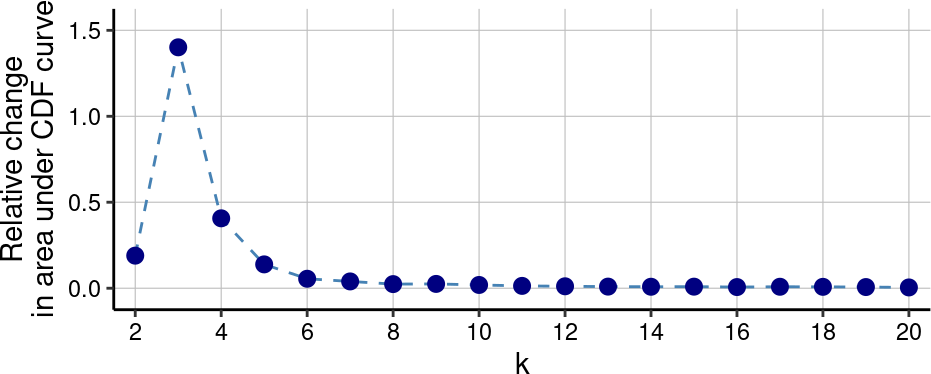# 4 Visualization

## 4.1plotCounts: Number of cells measured per sample

The number of cells measured per sample may be plotted with plotCounts. This plot should be used as a guide together with other readouts to identify samples where not enough cells were assayed. Here, the grouping of samples (x-axis) is controlled by group_by; bars can be colored by a an additional cell metadata variable (argument color_by):

plotCounts(sce,
group_by = "sample_id",
color_by = "condition")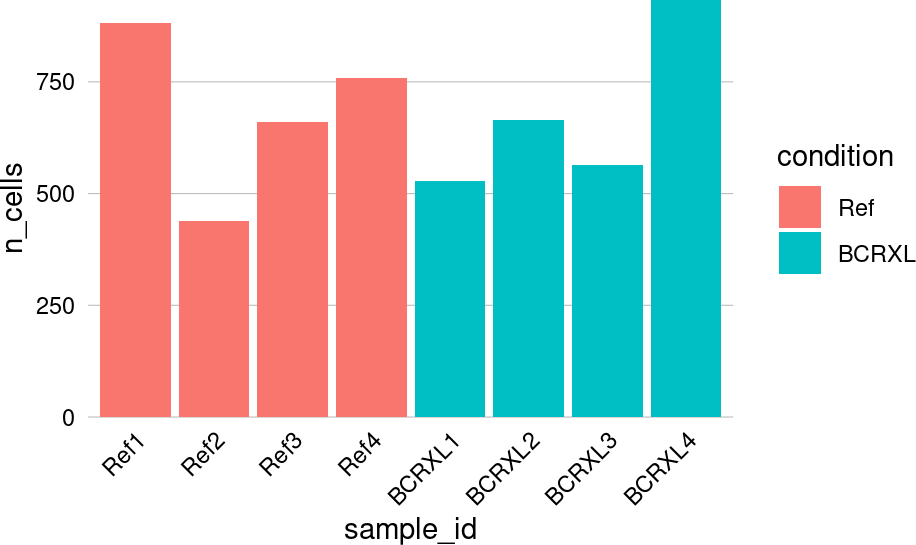As opposed to plotting absolute cell counts, argument prop can be used to visualize relative abundances (frequencies) instead:

plotCounts(sce,
prop = TRUE,
group_by = "condition",
color_by = "patient_id")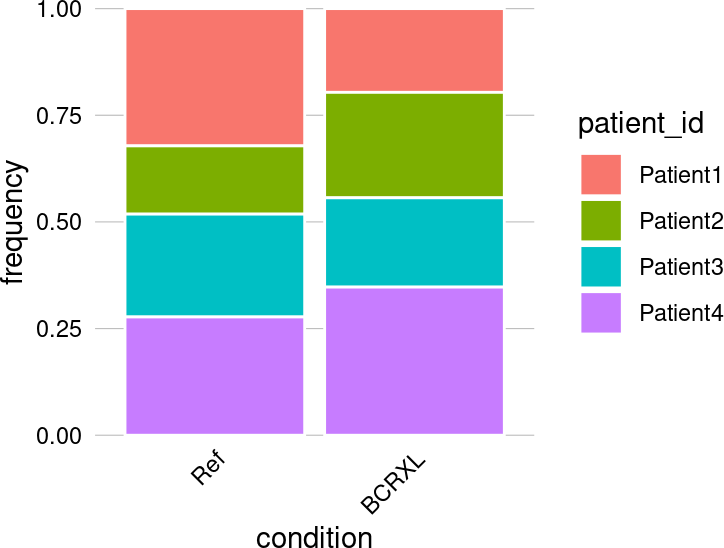## 4.2pbMDS: Pseudobulk-level MDS plot

A multi-dimensional scaling (MDS) plot on aggregated measurement values may be rendered with pbMDS. Such a plot will give a sense of similarities between cluster and/or samples in an unsupervised way and of key difference in expression before conducting any formal testing.

Arguments by, assay and fun control the aggregation strategy, allowing to compute pseudobulks by sample (by = "sample_id"), cluster (by = "cluster_id") or cluster-sample instances (by = "both") using the specified assay data and summarry statistic (argument fun)1 By default, median expression values are computed.. When by != "sample_id", i.e., when aggregating by cluster or cluster-sample, argument k specifies the clustering to use. The features to include in the computation of reduced dimensions may be specified via argument features.

Arguments color_by, label_by, shape_by can be used to color, label, shape pseudobulk instances by cell metadata variables of interest. Moreover, size_by = TRUE will scale point sizes proportional to the number of cells that went into aggregation. Finally, a custom color palette may be supplied to argument pal.

### 4.2.1 Ex. 2: MDS on sample-level pseudobulks

A multi-dimensional scaling (MDS) plot on median marker expression by sample has the potential to reveal global proteomic differences across conditions or other experimental metadata. Here, we color points by condition (to reveal treatment effects) and further shape them by patient (to highlight patient effects). In our example, we can see a clear horizontal (MDS dim. 1) separation between reference (REF) and stimulation condition (BCRXL), while patients are, to a lesser extent, separated vertically (MDS dim. 2):

pbMDS(sce, shape_by = "patient_id", size_by = FALSE)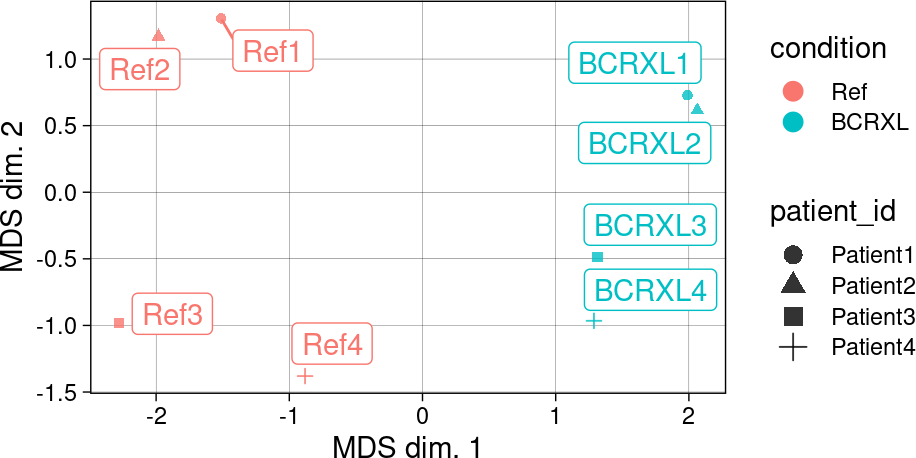### 4.2.2 Ex. 1: MDS on pseudobulks by cluster-sample

Complementary to the visualize above, we can generate an MDS plot on pseudobulks computed for each cluster-sample instance. Here, we color point by cluster (to highlight similarity between cell subpopulations), and shape them by condition (to reveal subpopulation-specific expression changes across conditions). In this example, we can see that cluster-sample instances of the same cell subpopulations group together. Meanwhile, most subpopulations exhibit a shift between instances where samples come from different treatment groups:

pbMDS(sce, by = "both", k = "meta12",
shape_by = "condition", size_by = TRUE)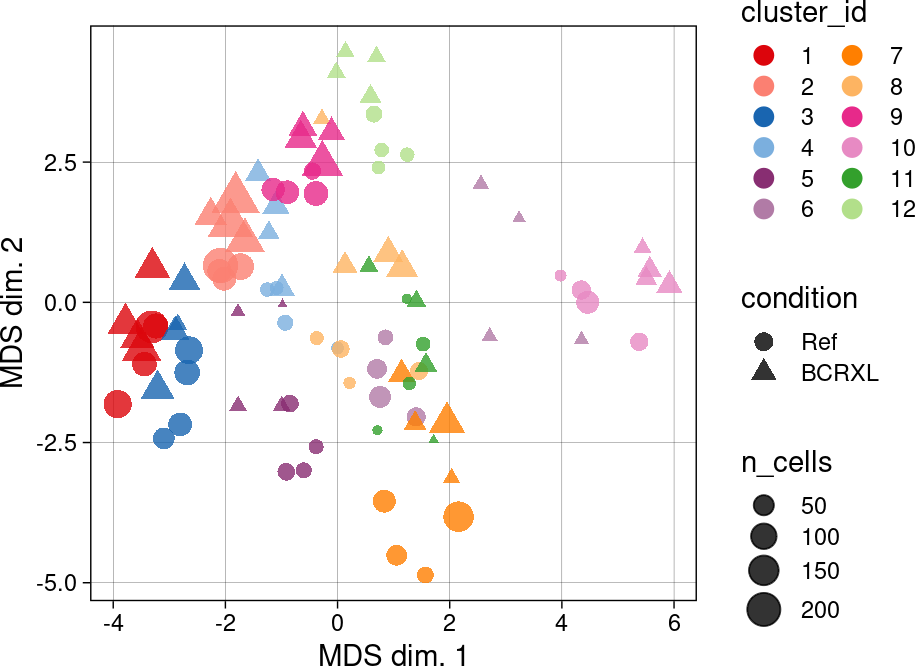## 4.3clrDR: Reduced dimension plot on CLR of proportions

A dimensionality reduction plot on centered log-ratios (CLR) of sample/cluster proportions across clusters/samples can be rendered with clrDR. Here, we view each sample (cluster) as a vector of cluster (sample) proportions. Complementary to pbMDS, such a plot will give a sense of similarities between samples/clusters in terms of their composition.

Centered log-ratio
Let $$s_i=s_1,...,s_S$$ denote one of $$S$$ samples, $$k_i=k_1,...,k_K$$ one of $$K$$ clusters, and $$p_k(s_i)$$ be the proportion of cells from sample $$s_i$$ in cluster $$k$$. The centered log-ratio (CLR) on a given sample’s cluster composition is then defined as:

$\text{clr}_{sk} = \log p_k(s_i) - \frac{1}{K}\sum_{i=1}^K \log p_k(s_i)$

Thus, each sample $$s$$ gives a vector with length $$K$$ with mean 0, and the CLRs computed across all instances can be represented as a matrix with dimensions $$S \times K$$.
We can embed the CLR matrix into a lower dimensional space in which points represent samples; or embed its transpose, in which case points represent samples. Distances in this lower-dimensional space will then represent the similarity in cluster compositions between samples and the in sample compositions between clusters, respectively.

Dimensionality reduction
In principle, clrDR allows any dimension reduction to be applied on the CLRs, with dims (default c(1, 2)) specifying which dimensions to visualize. The default method dr = "PCA" will include the percentage of variance explained by each principal component (PC) in the axis labels. Noteworthily, distances between points in the lower-dimensional space are meaningful only for linear DR methods (PCA and MDS), and results obtained from other methods should be interpreted with caution. The output plot’s aspect ratio should thus be kept as is for PCA and MDS; meanwhile, non-linear DR methods can use aspect.ratio = 1, rendering a squared plot.

For dr = "PCA", PC loadings will be represented as arrows that may be interpreted as follows: 0° (180°) between vectors indicates a strong positive (negative) relation between them, while vectors that are orthogonal to one another (90°) are roughly independent.
When a vector points towards a given quadrant, the variability in proportions for the points within this quadrant are largely driven by the corresponding variable. Here, only the relative orientation of loading vectors to each other and to the PC axes is meaningful; however, the sign of loadings (i.e., whether an arrow points left or right) can be flipped when re-computing PCs.

Aesthetics
Cell metadata variables to color points and PC loading arrows by are determined by arguments point/arrow_col, with point/arrow_pal specifying the color palettes to use for each layer. For example, rather than coloring samples by their unique identifiers, we may choose to use their condition for coloring instead to highlight differences across groups. In addition, point sizes may be scaled by the number of cells in a given sample/cluster (when by = "sample/cluster_id") via setting size_by = TRUE.
Argument arrow_len (default 0.5) controls the length of PC loading vectors relative to the largest absolute xy-coordinate. When specified, PC loading vectors will be re-scaled to improve their visibility: A value of 1 will stretch vectors such that the largest loading will touch on the outer most point. Importantly, while absolute arrow lengths are not interpretable, their relative length is.

### 4.3.1 Ex. 1: CLR on cluster proportions across samples

We here visualize the first two PCs computed on CLRs of sample proportions across clusters: small distances between samples mean similar cluster compositions between them, while large distances are indicative of differences in cluster proportions. In our example, PC 1 clearly separates treatment groups:

clrDR(sce, by = "sample_id", k = "meta12")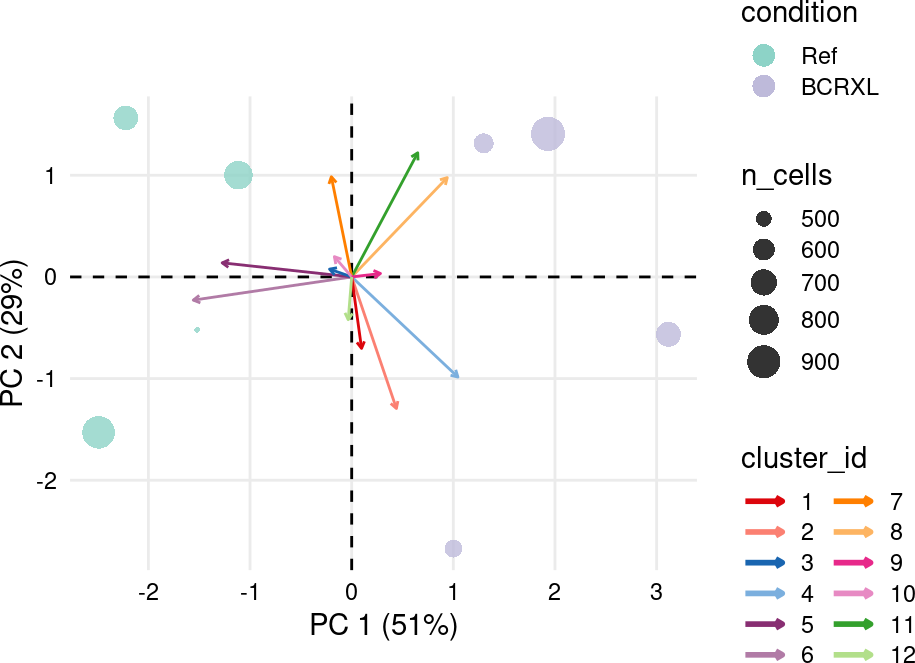### 4.3.2 Ex. 2: CLR on sample proportions across clusters

As an alternative to the plot above, we can visualize the first two PCs computed on the CLR matrix’ transpose. Here, we can observe that most of the variability in cluster-compositions across samples is driven by BCRXL samples (PC1):

clrDR(sce, by = "cluster_id", arrow_col = "condition", size_by = FALSE)